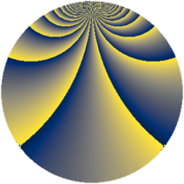# Properties

 Label 507.2.pLevel $507$ Weight $2$ Character orbit 507.p Rep. character $\chi_{507}(25,\cdot)$ Character field $\Q(\zeta_{26})$ Dimension $360$ Newform subspaces $2$ Sturm bound $121$ Trace bound $1$

# Related objects

## Defining parameters

 Level: $$N$$ $$=$$ $$507 = 3 \cdot 13^{2}$$ Weight: $$k$$ $$=$$ $$2$$ Character orbit: $$[\chi]$$ $$=$$ 507.p (of order $$26$$ and degree $$12$$) Character conductor: $$\operatorname{cond}(\chi)$$ $$=$$ $$169$$ Character field: $$\Q(\zeta_{26})$$ Newform subspaces: $$2$$ Sturm bound: $$121$$ Trace bound: $$1$$ Distinguishing $$T_p$$: $$2$$

## Dimensions

The following table gives the dimensions of various subspaces of $$M_{2}(507, [\chi])$$.

Total New Old
Modular forms 744 360 384
Cusp forms 696 360 336
Eisenstein series 48 0 48

## Trace form

 $$360q + 2q^{3} + 30q^{4} - 30q^{9} + O(q^{10})$$ $$360q + 2q^{3} + 30q^{4} - 30q^{9} - 4q^{10} - 6q^{12} - 50q^{13} - 8q^{14} - 14q^{16} + 8q^{17} - 56q^{22} - 4q^{23} + 14q^{25} + 12q^{26} + 2q^{27} - 4q^{29} - 4q^{30} - 52q^{31} + 130q^{32} - 130q^{34} + 4q^{35} + 30q^{36} + 138q^{38} - 2q^{39} + 12q^{40} - 118q^{42} - 4q^{43} - 2q^{48} - 126q^{49} - 16q^{51} + 128q^{52} - 74q^{53} + 90q^{55} - 24q^{56} - 52q^{58} - 208q^{59} + 104q^{60} + 20q^{61} + 102q^{62} + 10q^{64} - 88q^{66} + 52q^{67} - 118q^{68} - 4q^{69} - 78q^{71} + 88q^{74} + 2q^{75} - 260q^{76} + 8q^{77} + 118q^{78} + 28q^{79} - 30q^{81} + 86q^{82} + 78q^{85} + 156q^{86} - 88q^{87} - 296q^{88} - 4q^{90} - 128q^{91} + 28q^{92} - 130q^{93} + 50q^{94} - 124q^{95} + 156q^{97} + O(q^{100})$$

## Decomposition of $$S_{2}^{\mathrm{new}}(507, [\chi])$$ into newform subspaces

Label Dim. $$A$$ Field CM Traces $q$-expansion
$$a_2$$ $$a_3$$ $$a_5$$ $$a_7$$
507.2.p.a $$168$$ $$4.048$$ None $$0$$ $$-14$$ $$0$$ $$0$$
507.2.p.b $$192$$ $$4.048$$ None $$0$$ $$16$$ $$0$$ $$0$$

## Decomposition of $$S_{2}^{\mathrm{old}}(507, [\chi])$$ into lower level spaces

$$S_{2}^{\mathrm{old}}(507, [\chi]) \cong$$ $$S_{2}^{\mathrm{new}}(169, [\chi])$$$$^{\oplus 2}$$